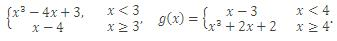×#### Thank you for registering.

One of our academic counsellors will contact you within 1 working day.

Click to Chat

1800-1023-196

+91-120-4616500

CART 0

• 0

MY CART (5)

Use Coupon: CART20 and get 20% off on all online Study Material

ITEM
DETAILS
MRP
DISCOUNT
FINAL PRICE
Total Price: Rs.

There are no items in this cart.
Continue Shopping```Algebra of FunctionsGiven functions f : D → R and g : D → R, we describe functions f + g, f - g, gf an f/g as follows:

f + g : D → R is a function defined by (f + g) (x) = f(x) + g(x)

f - g : D → R is a function defined by (f - g)(x) = f(x) - g(x)

fg : D → R is a function defined by (fg) (x) = f(x) g(x)

f/g : C → R is a function defined by (f/g) (x) = f(x)/g(x) , g(x) ≠ 0,

where C = {x ε D : g(x) ≠ 0}.

Illustration: Let f : [-1, 1] → R and g : R → R be functions defined by f(x) = √(1-x2) and g(x) = x3 + 1. Find the domain of f + g, fg and f/g.

Solution:

(f+g)(x) = f(x)+g(x) = √(1-x2) + x3 + 1, (f-g)(x) - g(x) = √(1-x2) - x3 = 1,

(fg)(x) = f(x) g(x) = (x3 + 1) √(1-x2)  and  (f/g)(x) = f(x)/g(x)  = (√(1-x2))/(x3- 1), x ≠ 1.

The domain of each of f + g. f - g and fg is [-1, 1] and of f/g is

{x ε [-1, 1] : g(x) ≠ 0} = (-1, 1].

Illustration: Let f(x) =Describe the function f/g.

Solution:

We redefine the functions f(x) and g(x) in the intervals as shown below:At askIITians we provide you free study material on Set Theory and Functions and you get all the professional help needed to get through IIT JEE and AIEEE easily. AskIITians also provides live online IIT JEE preparation and coaching where you can attend our live online classes from your home!

To read more, Buy study materials of Set Relations and Functions comprising study notes, revision notes, video lectures, previous year solved questions etc. Also browse for more study materials on Mathematics here.
```### Course Features

• 731 Video Lectures
• Revision Notes
• Previous Year Papers
• Mind Map
• Study Planner
• NCERT Solutions
• Discussion Forum
• Test paper with Video Solution